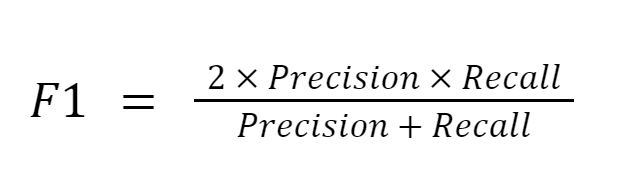# F1 score

Encord Computer Vision Glossary

F1 Score is a performance metric commonly used in binary classification problems. It is the harmonic mean of precision and recall, which measures the model's accuracy and completeness. F1 score ranges from 0 to 1, where 1 is the best possible score.

F1 score is a crucial performance metric in evaluating the effectiveness of a binary classification model. It measures the balance between the model's precision and recall, providing a more comprehensive evaluation of the model's performance. F1 score is often used in situations where precision and recall have equal importance, and a single score is needed to evaluate the model's overall performance.

To calculate the F1 score, one must first calculate precision and recall. Precision is the ratio of true positive (TP) to the sum of true positive and false positive (FP), while recall is the ratio of true positive to the sum of true positive and false negative (FN). F1 score is calculated as the harmonic mean of precision and recall, and its value ranges from 0 to 1.F1 score is widely used in various machine learning applications, including sentiment analysis, fraud detection, and medical diagnosis. It is often used with other performance metrics such as accuracy, precision, and recall to comprehensively evaluate the classification model's performance.One platform for creating better training data and debugging models.

In summary,

• F1 score is a crucial performance metric in binary classification problems
• Measures the model's balance between precision and recall
• Provides a comprehensive evaluation of the classification model's performance.
• Widely used in various machine learning applicationsGet our newsletter to learn about the latest developments in computer vision

###### Subscribe